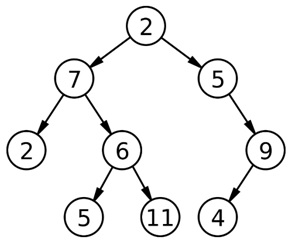# Find the level in a binary tree with given sum K

Here, we are going to learn how to find the level in a binary tree with given sum K – its an interview coding problem came in Samsung, Microsoft?
Submitted by Radib Kar, on November 25, 2018

Description:

The article describes how to find the level in a binary tree with given sum K? This is an interview coding problem came in Samsung, Microsoft.

Problem statement:

Given a binary tree and an integer K, output the level no of the tree which sums to K. Assume root level is level 0.

Solution

Algorithm:

One of the popular traversal techniques to solve this kind of problems is level order tree traversal (Read more: Level Order Traversal on a Binary Tree) where we use the concept of BFS with some modifications.

1. Set a variable level=0 & declare a variable of type tree* named temp. level is a flag for the current level & temp stores tree node to be processed.

2. Set cur_sum=0

3. if(!root) // root is NULL
return -1; //no such level exists

4. q=createQueue() //to store pointers to tree node

5. EnQueue(q,root);

6. EnQueue(q,NULL);

Every time, we EnQueue a NULL to reflect the end of current level. Same way while processing when NULL is found, it reveals that end of current level.

```7. while( q is not empty)
temp=DeQueue(q);
if(temp==NULL){ //end of current level
if (cur_sum==K) // current level sum is equal to K
Return level; //return level no (root is at 0 level)
else {
Set cur_sum =0; // for next level
If(q is not empty)
// to reflect end of next level which
// will be processed in next iteration
EnQueue(q,NULL)
Increase level //increase level count
}
}
Else{
cur_sum+=temp->data; //sum the level
//do level order traversal
If(temp->left)
EnQueue(temp->left);
If(temp->right)
EnQueue (temp->right);
}
End while loop
```

8. If program control comes out of while loop then surely no level has a sum K. Thus print no level has sum KExample:

```Here the level sums are:
For level 0: 2
For level 1: 12
For level 2: 17
For level 3: 20
Thus if K is 12 our program will print level 1
```

## C++ code to find the level in a binary tree with given sum K

```#include <bits/stdc++.h>
using namespace std;

// tree node is defined
class tree{
public:
int data;
tree *left;
tree *right;
};

int findLevel( tree *root,int K){
queue<tree*> q;  // using stl
tree* temp;
//counting level no & initializing cur_sum
int level=0,cur_sum=0;
//EnQueue root
q.push(root);
//EnQueue NULL to inticate end of 0 level
q.push(NULL);
while(!q.empty()){
//DeQueueing using STL
temp=q.front();
q.pop();
if(temp==NULL){
//if current level sum equals to K
if(cur_sum==K)
return level;//return level no
//ifn't then set cur_sum to 0 for further levels
cur_sum=0;
if(!q.empty())
q.push(NULL);
level++;
}
else{
//level order traversal
cur_sum+=temp->data; //sum thd level
if(temp->left)
q.push(temp->left); //EnQueue
if(temp->right)
q.push(temp->right); //EnQueue
}
}
return -1;
}

// creating new node
tree* newnode(int data)
{
tree* node = (tree*)malloc(sizeof(tree));
node->data = data;
node->left = NULL;
node->right = NULL;

return(node);
}

int main()
{
//**same tree is builted as shown in example**
int c,K;
cout<<"Tree is built like the example aforesaid"<<endl;
cout<<"input K....."<<endl;
cin>>K;

tree *root=newnode(2);
root->left= newnode(7);
root->right= newnode(5);
root->right->right=newnode(9);
root->right->right->left=newnode(4);
root->left->left=newnode(2);
root->left->right=newnode(6);
root->left->right->left=newnode(5);
root->left->right->right=newnode(11);

cout<<"finding if any level exists......"<<endl;
c=findLevel(root,K);
if(c>=0)
cout<<"level is found and it is level "<<c<<endl;
else

return 0;
}
```

Output

```First run:
Tree is built like the example aforesaid
input K.....
20
finding if any level exists......
level is found and it is level 3

Second run:
Tree is built like the example aforesaid
input K.....
25
finding if any level exists......
```